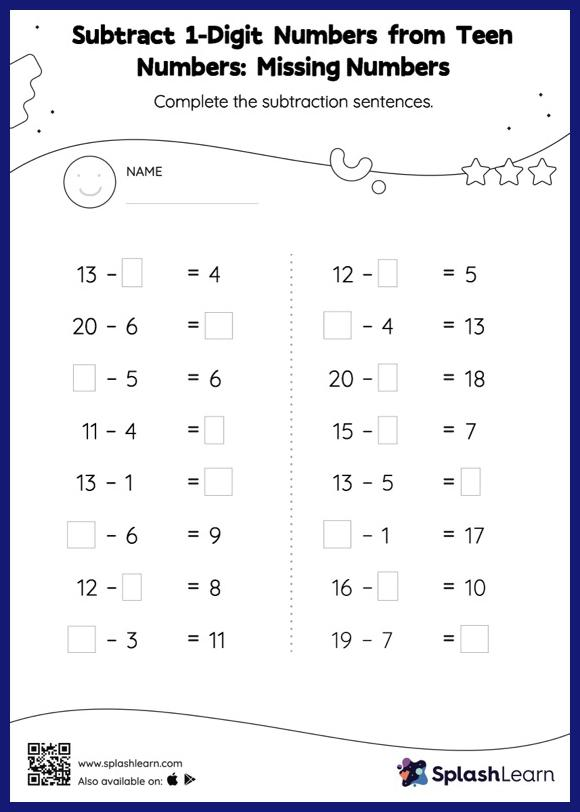# Subtract 1-Digit Numbers from Teen Numbers: Missing Numbers Worksheet

Home > Subtract 1-Digit Numbers from Teen Numbers: Missing NumbersDoes your child know how to subtract 1-digit numbers from teen numbers? Invite them to practice this concept here. In this worksheet, students must find the missing number using the count back method or the relationship between addition and subtraction. To solve subtract 1-digit numbers from teen numbers worksheet, they do not need to regroup the numbers. As the worksheet uses a horizontal format, it allows for more creativity when it comes to how the student solves the problem. In contrast, in the vertical format, students usually employ the standard method to solve.